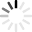# Circle Quizzes & Trivia

Round and round and round we go, where we stop, nobody knows! Actually, we do... Next stop, geometry quizzes! One of the most basic shapes we know since childhood is the "circle". In geometry though, the circle has a lot of properties and equations associated with it. Are you in shape to answer our quizzes on circles? First, why don't you try out some terminologies involving circles.

Arc, center, diameter, radius; are you any good at this "sector"? How about some problems on area and circumference? Do you think you can make it around these questions? We have questions on different properties and theorems too. Stop going in circles and check out our quizzes. Worrying too much, are you? Relax and get on with the quizzes. We promise, it's going to be as easy as "pi"!
Top Trending

Yes, the circle has parts! A circle may seem the same at all points but the quiz below will enlighten you on identifying the parts of a circle. It is a challenge worth a shot. Enjoy the discovery!

Questions: 5  |  Attempts: 6227   |  Last updated: Sep 10, 2020
• Sample Question
Which of the following is a chord, but not a diameter?Can you pass this Circumference and Area of Circle Quiz? Some students get confused identifying which formulas are used when it comes to getting the circumference or area of a circle. The confusion comes in knowing which one to...

Questions: 15  |  Attempts: 2905   |  Last updated: Sep 15, 2020
• Sample Question
Round to the nearest tenth. Find the circumference of a circle with a radius of 5.6 meters.Working out angles in circles

Questions: 20  |  Attempts: 585   |  Last updated: Dec 30, 2020

A circle is a shape consisting of all points in a plane that are at a given distance from a given point, centre. The equation of circle is an important mathematical topic in the chapter of circles, and is a must to prepare for...

Questions: 5  |  Attempts: 1309   |  Last updated: Apr 15, 2021
• Sample Question
Find the equation of the circle with centre (-1, 2) and radius = 2 units.A circle is a closed two-dimensional figure in which the set of all the points in the plane is equidistant from a given point called “centre”. Every line that passes through the circle forms the...

Questions: 16  |  Attempts: 422   |  Last updated: Apr 15, 2021Related